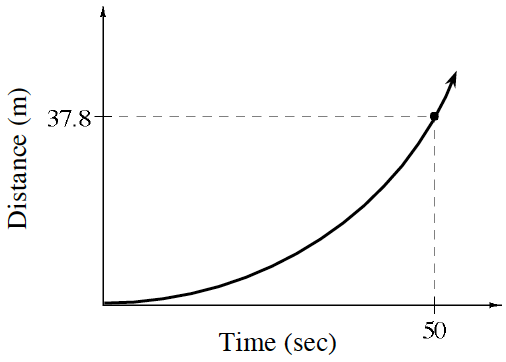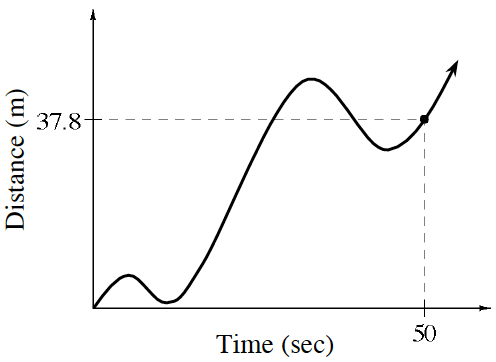### Home > APCALC > Chapter 3 > Lesson 3.2.3 > Problem3-79

3-79.

Calculate the average velocity between $0$ and $50$ seconds for each of the graphs below.

1.$\text{Average velocity} = \frac{\Delta \text{distance}}{\Delta \text{time}}$

1.This graph shows both forward and backwards motion. Will that affect average velocity?